#【深度学习之美】山重水复疑无路，最快下降问梯度（入门系列之七）

0
1
01. 云栖社区>
2. 博客>
3. 正文

## 【深度学习之美】山重水复疑无路，最快下降问梯度（入门系列之七）

### 系列文章：

1986年，辛顿教授和他的小伙伴们重新设计了BP算法，以“人工神经网络”模仿大脑工作机理，“吻”醒了沉睡多年的“人工智能”公主，一时风光无限。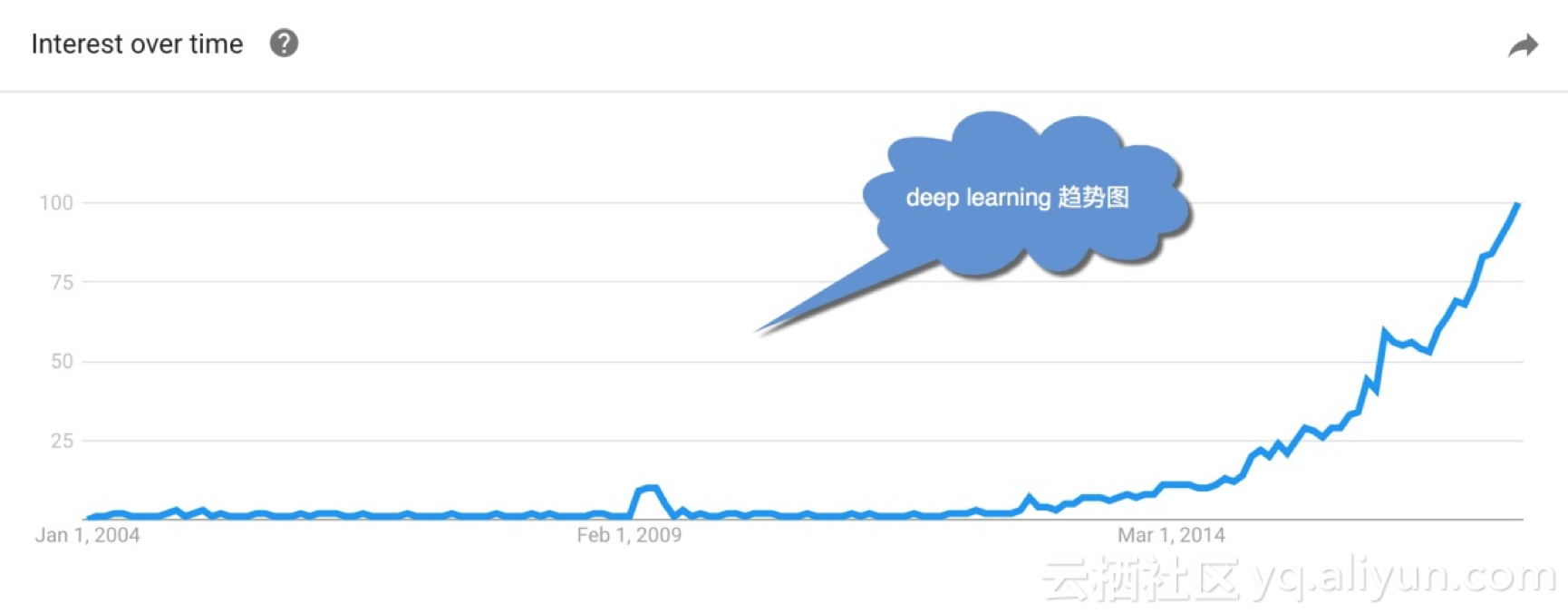## 7.1 1986年的那篇神作

1986年10月，杰弗里•辛顿还在卡内基梅隆大学任职。他和在加州大学圣迭戈分校的认知心理学家大卫·鲁梅尔哈特（David Rumelhart）等人，在著名学术期刊《自然》上联合发表题为：“通过反向传播算法的学习表征（Learning Representations by Back-propagating errors）”的论文。该文首次系统简洁地阐述反向传播算法（BP）在神经网络模型上的应用，该算法把网络权值纠错的运算量,从原来的与神经元数目的平方成正比，下降到只和神经元数目本身成正比。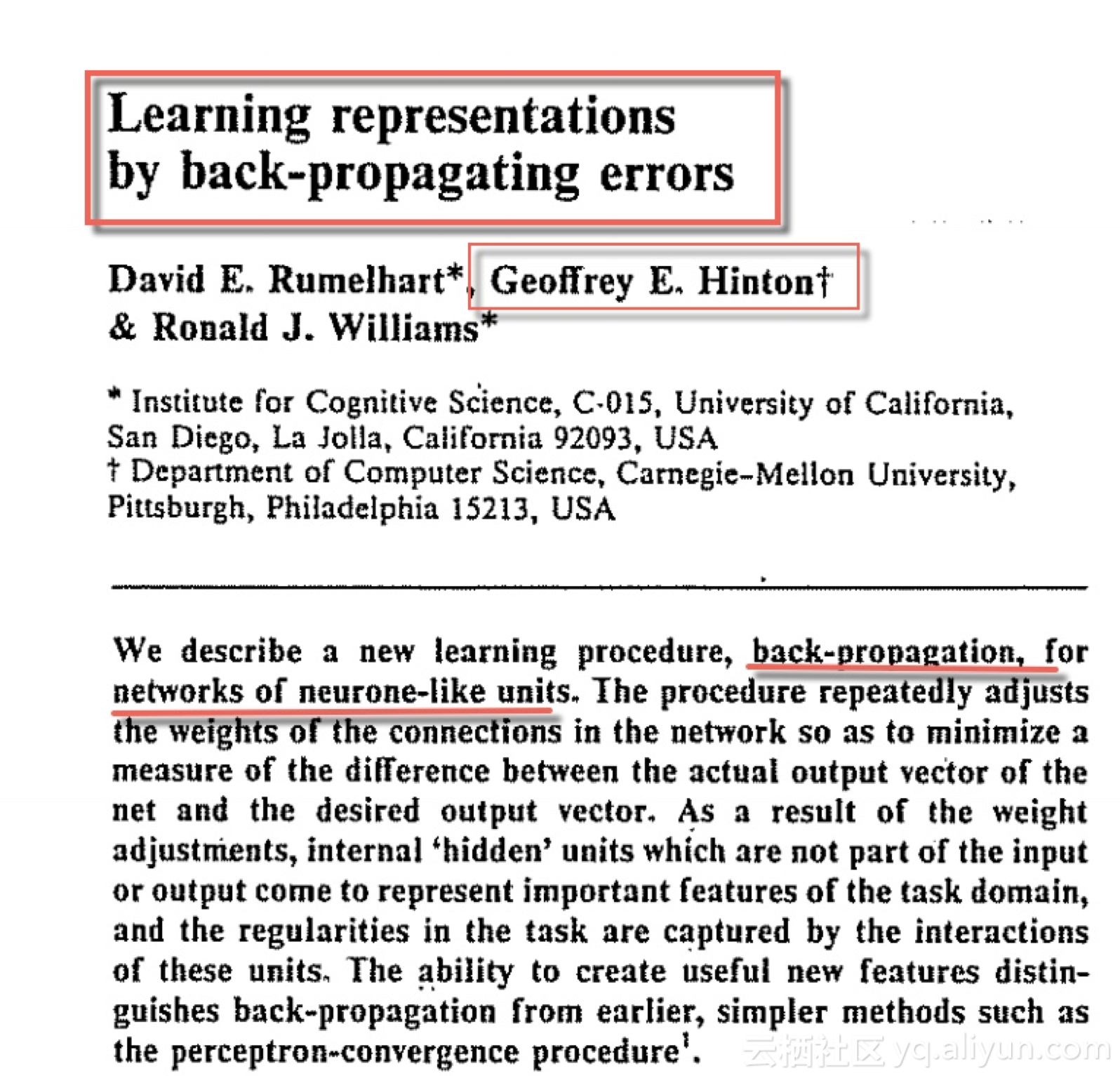## 7.2 多层感知机网络遇到的大问题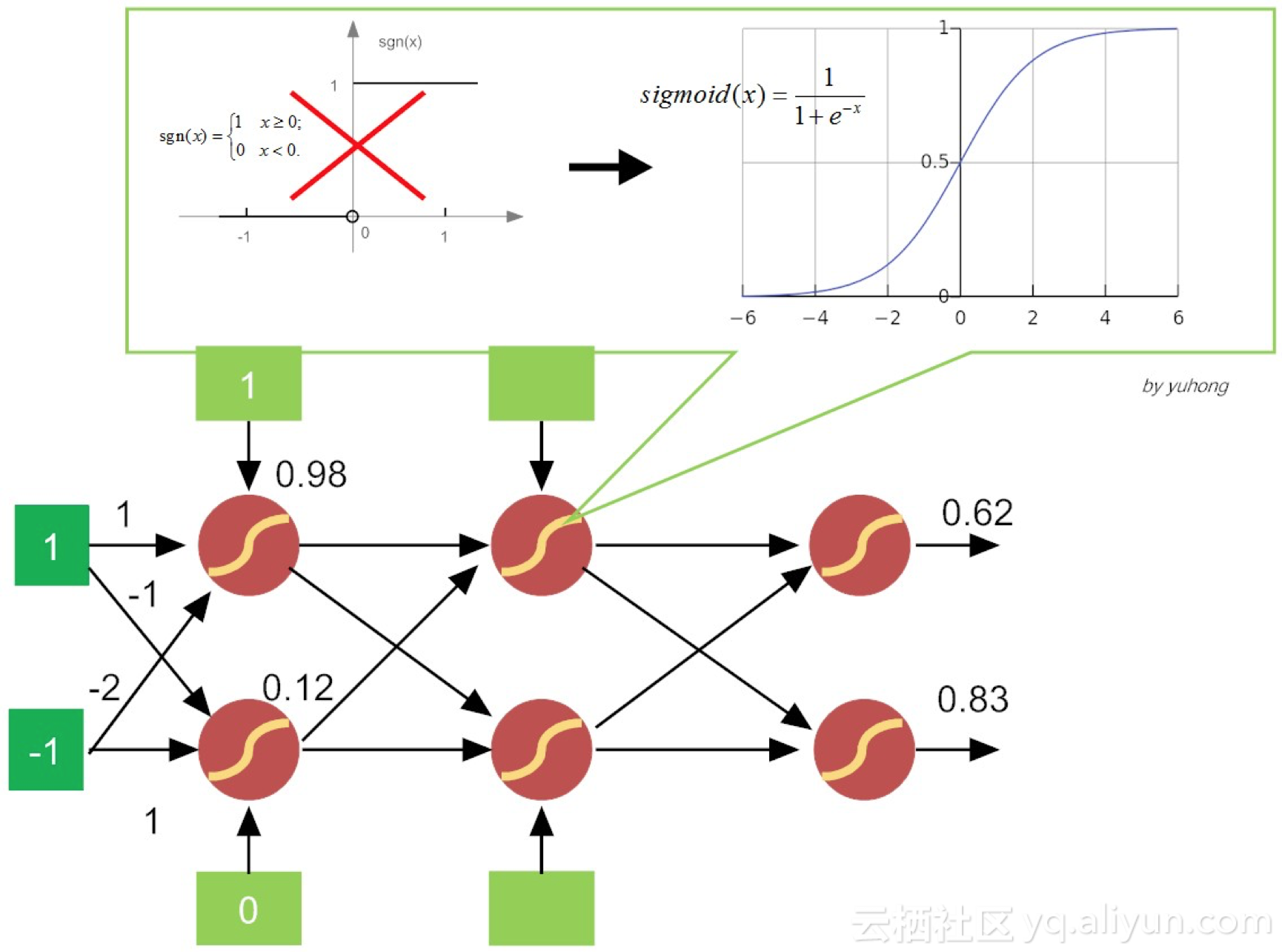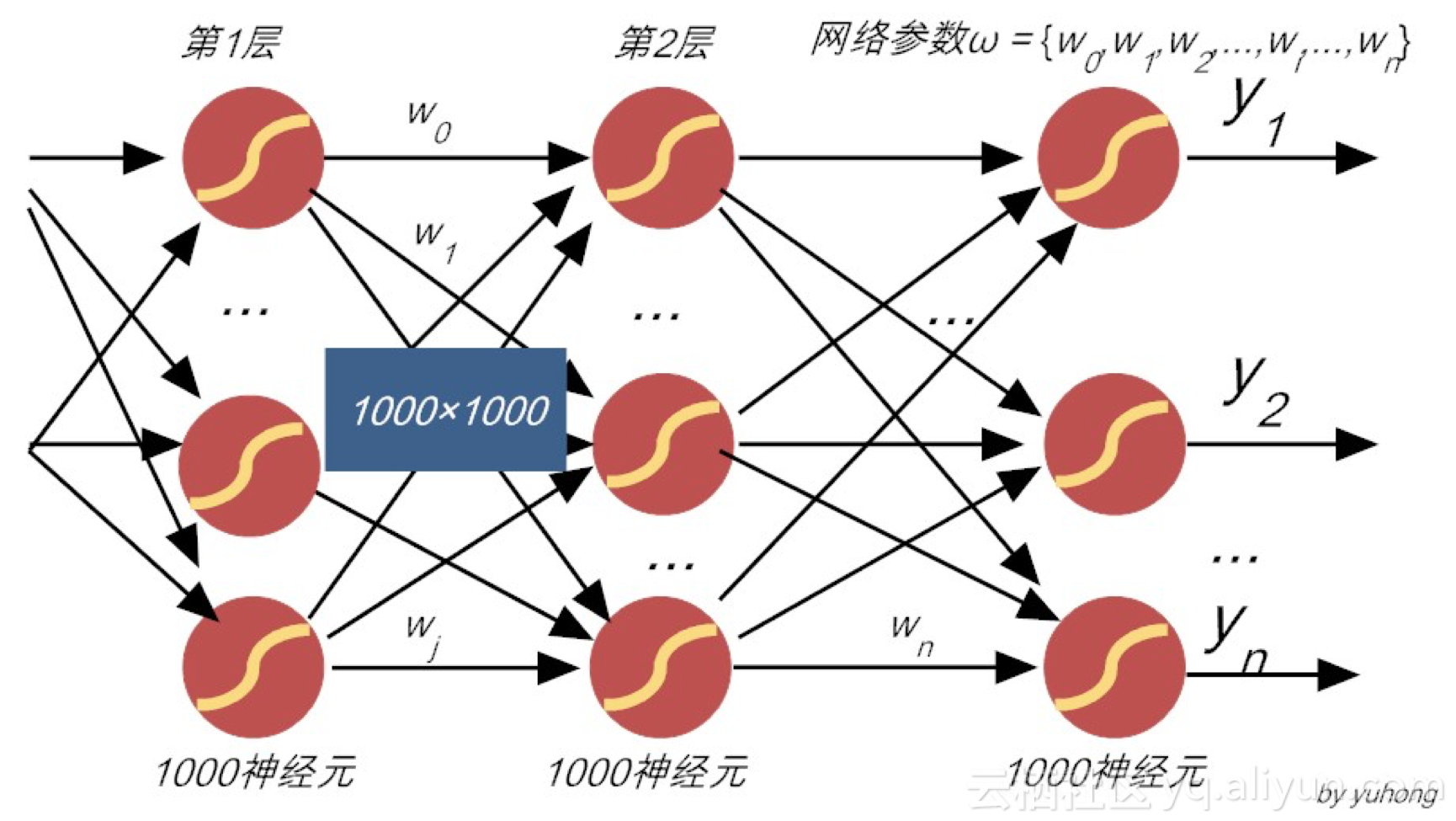## 7.3到底什么是梯度？

delta法则的核心思想在于，使用梯度下降（gradient descent）的方法找极值。具体说来，就是在假设空间中搜索可能的权值向量，并以“最佳”的姿态，来拟合训练集合中的样本。那么，何谓最佳拟合呢？当然就是让前文提到的损失函数达到最小值！

$$f'({x_0}) = \mathop {\lim }\limits_{\Delta x \to 0} \frac{{\Delta y}}{{\Delta x}} \\\\ = \mathop {\lim }\limits_{\Delta x \to 0} \frac{{f({x_0} + \Delta x) - f({x_0})}}{{\Delta x}}\tag{7.1}$$

$$\nabla f = \left( {\frac{{\partial f}}{{\partial x}},\frac{{\partial f}}{{\partial y}},\frac{{\partial f}}{{\partial z}}} \right)\tag{7.2}$$

（1） 把$$y$$，$$z$$视为常量，得$$x$$的“局部导数”：

$$\frac{{\partial f}}{{\partial x}} = 2x + 3y$$

（2） 然后把$$x$$，$$z$$视为常量，得$$y$$的“局部导数”：

$$\frac{{\partial f}}{{\partial y}} = 3x + 2y$$

（3） 最后把$$x$$，$$y$$视为常量，得$$z$$的“局部导数”：

$$\frac{{\partial f}}{{\partial y}} = 3{z^2}$$

$$\nabla f = grad(f) \\\\ = \left( {2x + 3y,3x + 2y,3{z^2}} \right)$$

\begin{aligned} \nabla f & = grad(f)\\\\&=(2x+3y,3x+2y,3z^2)\mid_{\begin{aligned}& x=1 \\\\ & y=2 \\\\ & z=3 \end{aligned}} \\\\ & = (8,7,27) \end{aligned}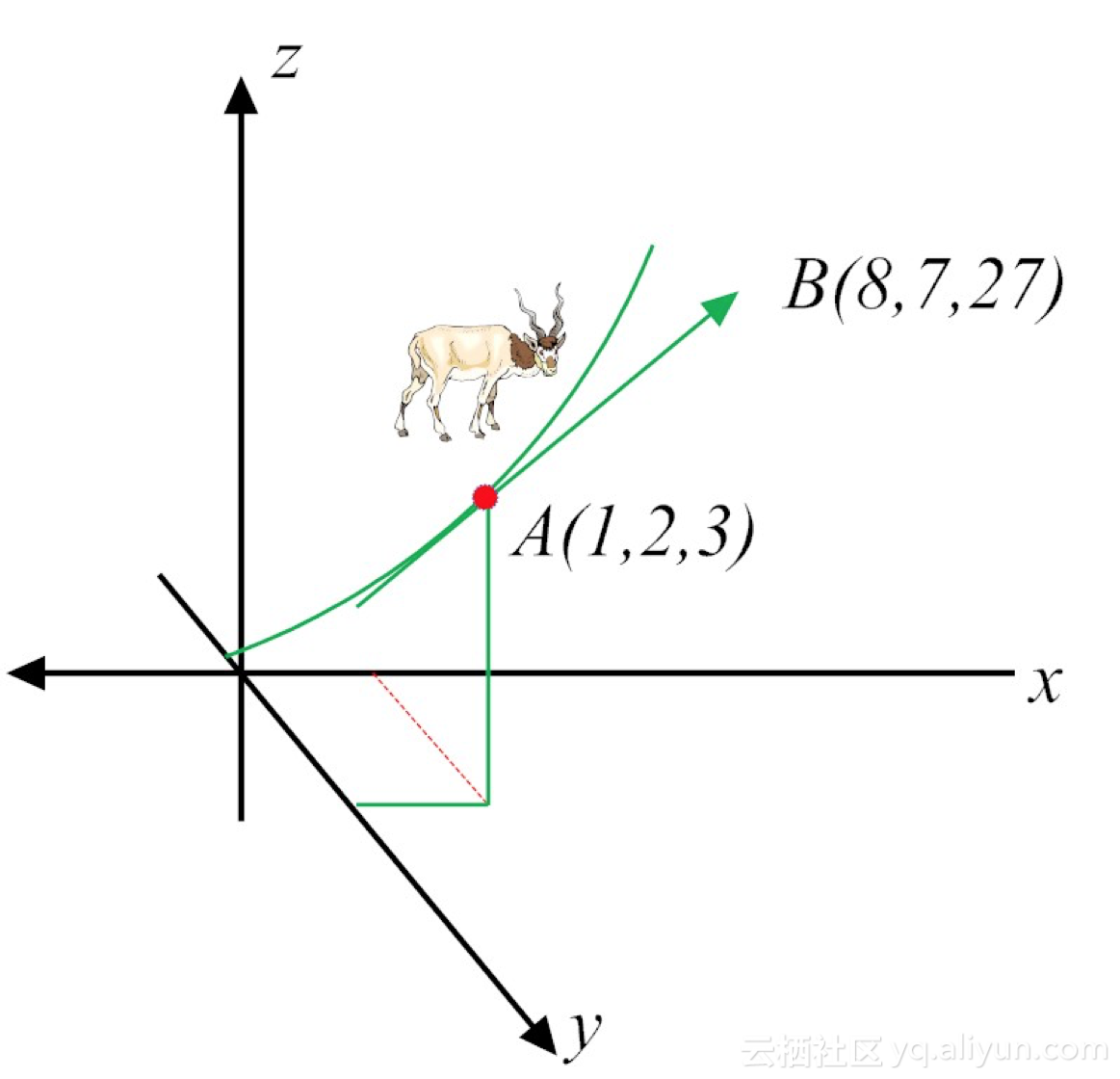## 7.4 到底什么是梯度下降？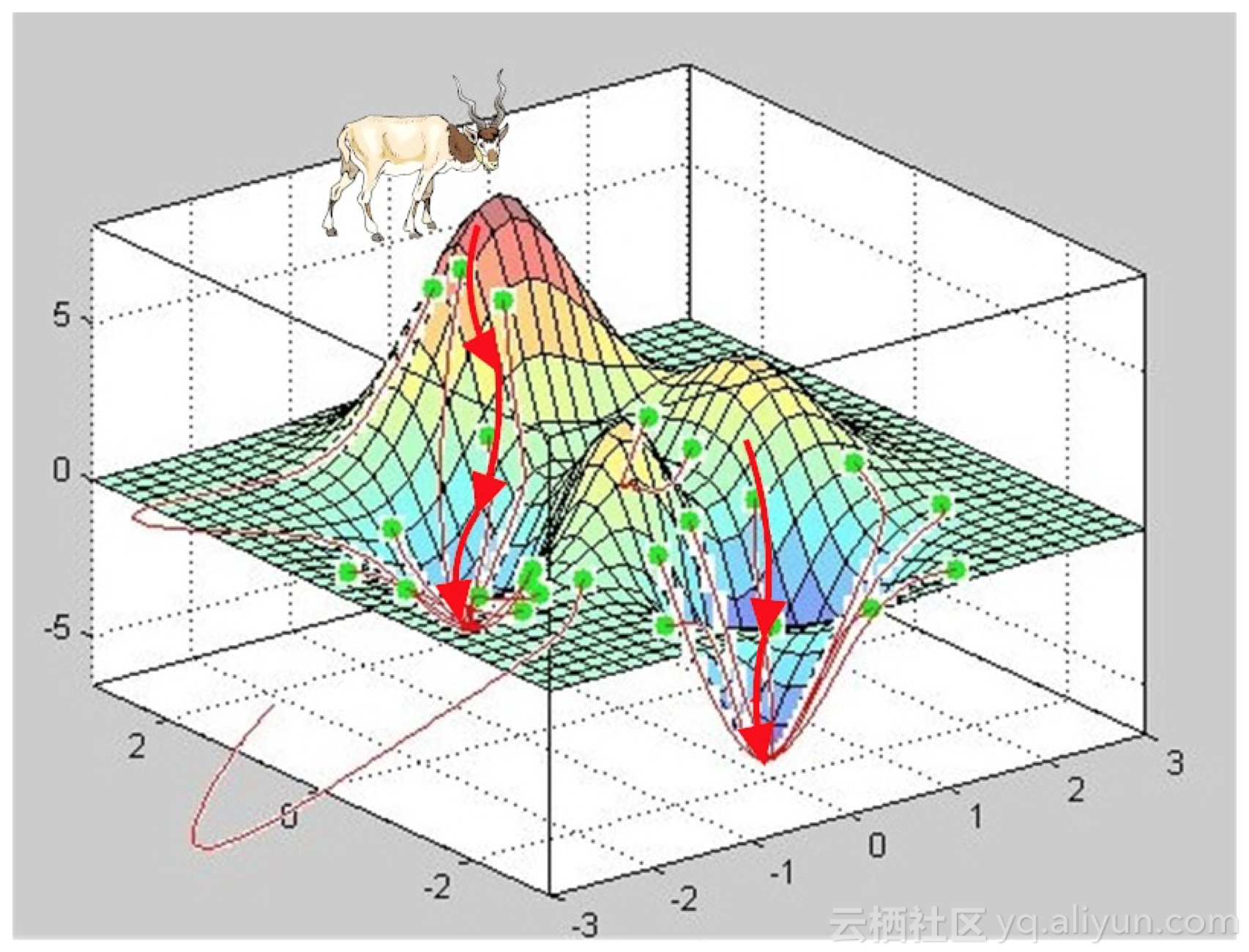## 7.5 重温神经网络的损失函数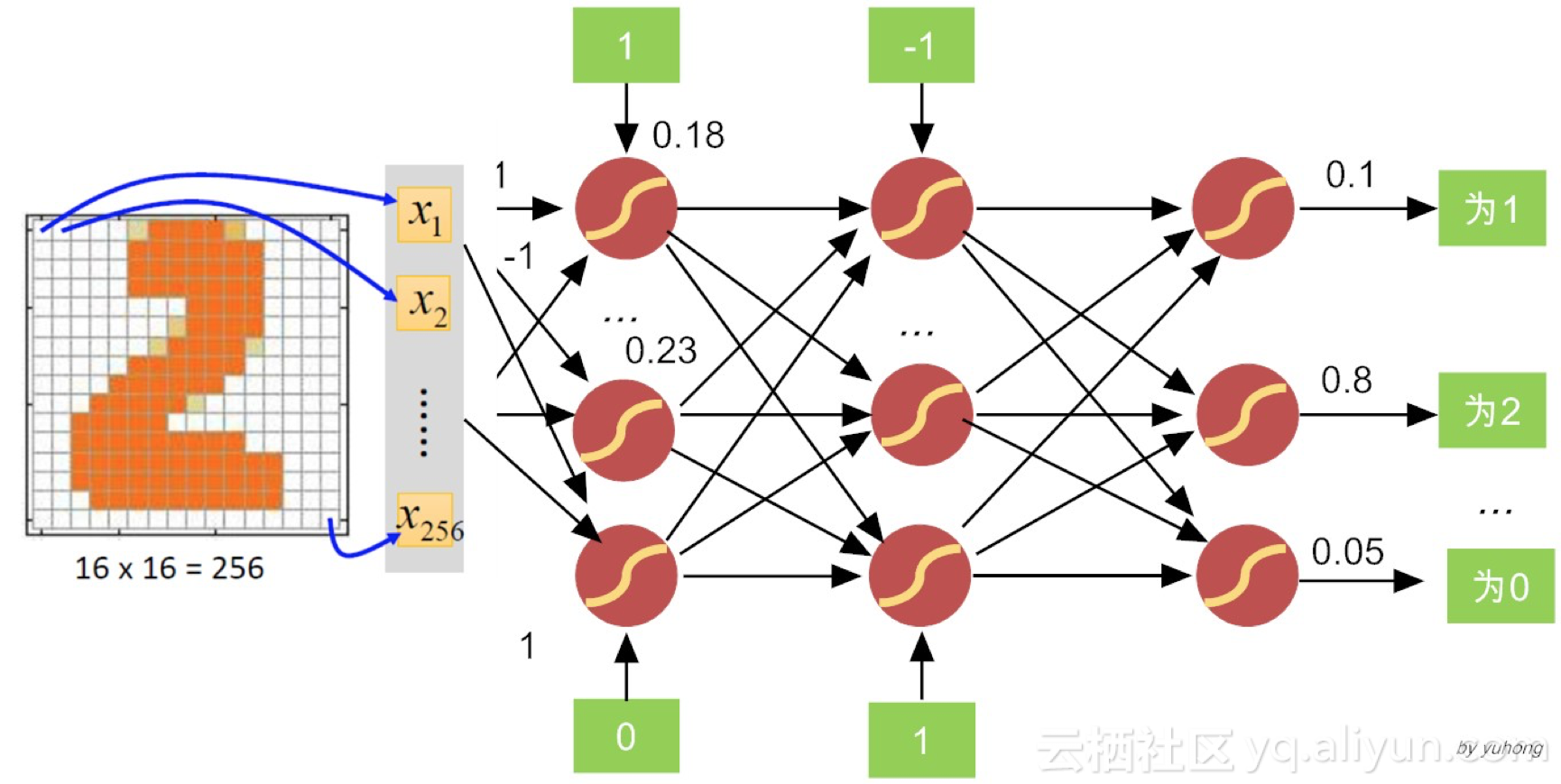$$(Y,f(X)) = \frac{1}{2}{(Y - f(X))^2}\tag{7.3}$$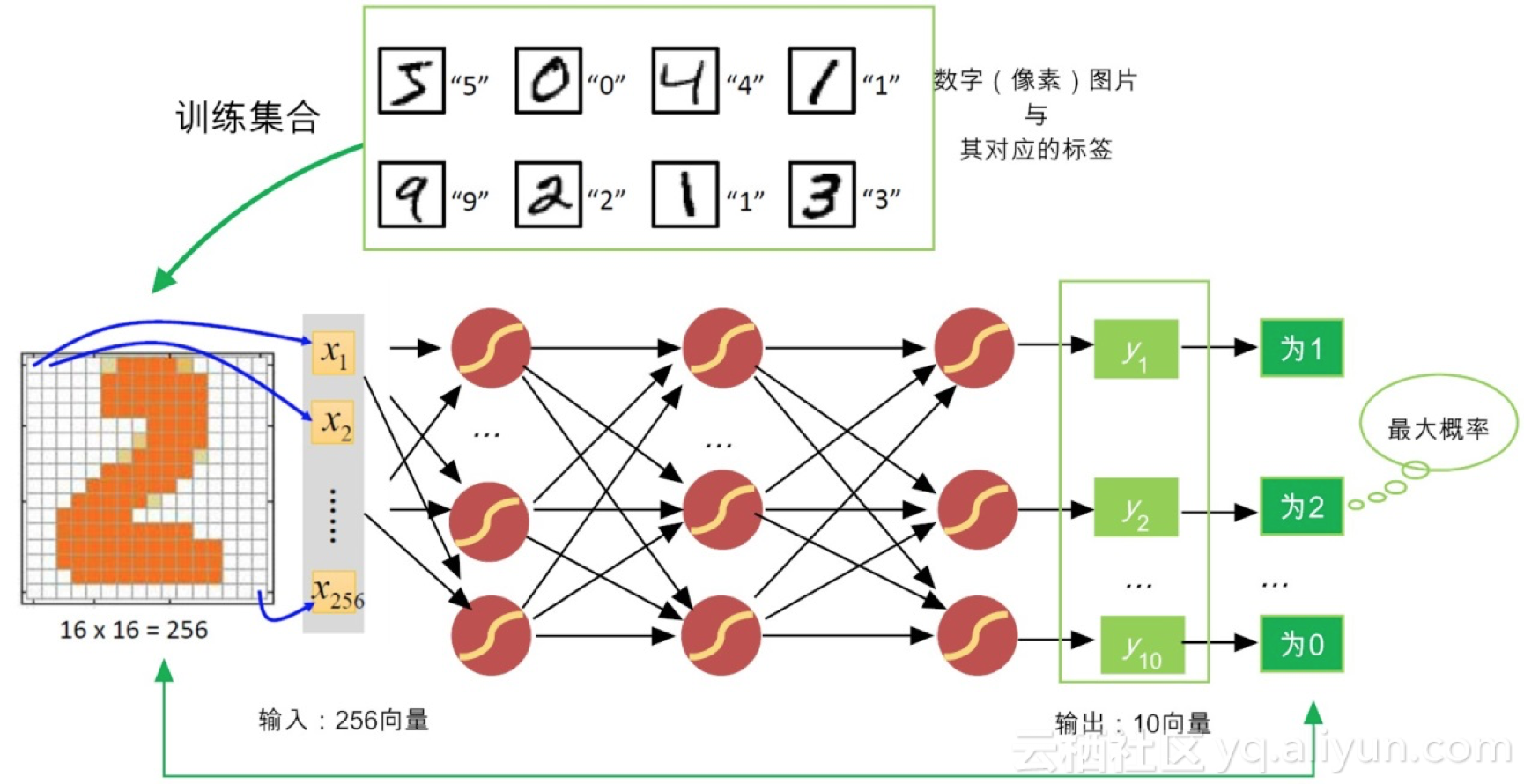$$\nabla L(\mathop w\limits^ \to ) \equiv \left[ {\frac{{\partial L}}{{\partial {w_0}}},\frac{{\partial L}}{{\partial {w_2}}},...,\frac{{\partial L}}{{\partial {w_n}}}} \right]\tag{7.4}$$

\begin{aligned} \frac{\partial L}{\partial w_{i}}= & \frac{\partial}{\partial w_{i}}\frac{1}{2}(Y-f(X))^2=\frac{1}{2}\sum_{d\in D}\frac{\partial}{\partial w_{i}}(y_{d}-y_{d}')^2 \\\\ = & \frac{1}{2}\sum_{d\in D}2(y_{d}-y_{d}')\frac{\partial}{\partial w_{i}}(y_{d}-y_{d}') \\\\ = & \sum_{d\in D}(y_{d}-y_{d}')\frac{\partial}{\partial w_{i}}(y_{d}-\vec{w}\cdot\vec{x}_{d}) \\\\ = & \sum_{d\in D}(y_{d}-y_{d}')(y_{d}-\vec{x}_{id}) \end{aligned} \tag{7.5}

## 7.6 小结

“世上没有白走的路，每一步都算数”。希望你能持续关注。

## 7.7 请你思考

（1）在前馈神经网络中，隐含层设计多少层、每一层有多少神经元比较合适呢？我们可以设定一种自动确定网络结构的方法吗？
（2）神经网络具有强大的特征表征能力，但“成也萧何，败也萧何”，BP算法常常遭遇“过拟合（overfitting）”，它可能会把噪音当做有效信号，你知道有什么策略来避免过拟合吗？

## 【参考文献】

 吴军. 智能时代. 中信出版集团. 2016.8
 Hinton G E, Osindero S, Teh Y W. A fast learning algorithm for deep belief nets[J]. Neural computation, 2006, 18(7): 1527-1554.
 Williams D, Hinton G. Learning representations by back-propagating errors[J]. Nature, 1986, 323(6088): 533-538.
 Rumelhart D E, McClelland J L, PDP Research Group. Parallel Distributed Processing, Volume 1 Explorations in the Microstructure of Cognition: Foundations[J]. 1986.
 Tom Mitchell著.曾华军等译. 机器学习. 机器工业出版社. 2007.4
 Better Explained. Vector Calculus: Understanding the Gradient

## 【参考文献】

 (美) 雷·库兹韦尔, 李庆诚等译. 奇点临近.机械工业出版社.2012.12
2尤瓦尔·赫拉利，未来简史. 出版社：中信出版社.2017.1
 李航.统计学习方法.清华大学出版社.2012.3
 Hornik K, Stinchcombe M, White H. Multilayer feedforward networks are universal approximators[J]. Neural networks, 1989, 2(5): 359-366.

##（未完待续）

+ 关注